# Proportions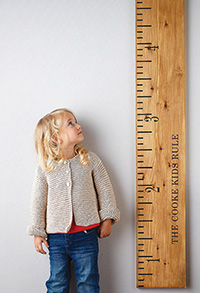In this lesson, you will learn to write proportions and solve problems using proportions. You will also learn to use the properties of similar figures and proportions to solve for unknown lengths, perimeters, and areas.

First, we need to look at how units are used in our ratio problems. Take a look at the problem below.

Heather knew that she was 60 inches tall and that her younger brother, Ryan, was 5 feet tall. She claimed that she was 12 times as tall as Ryan because 5 times 12 is 60. Ryan disagreed with Heather and claimed that they were the same height. Can you see how important it is to label your units when they are different?

Remember that a ratio is the comparison of two quantities by division. A ratio can be written as a fraction, in words, or with colon notation. Please use the fraction form in this class so we can properly set up and solve problems.

•• 22 miles to 1 gallon
• 22 miles : 1 gallon

A ratio should never be written as a mixed number and must have units labeled when these units are different.

The way that a ratio is written tells which value is written as the numerator and which is the denominator. For example, if you compare 60 miles to 1 hour, you will write these values as. The units in a ratio help you remember the order in which to write it, especially when you are comparing ratios. If you are comparing the same types of things, you do not have to use the units. For example, if you compare 12 feet to 8 feet, you can write. You can simplify the fractions to make comparisons easier, but remember not to use mixed numbers. This ratio will simplify to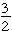.

Try writing a few on your own to make sure you understand the concept.

## Writing Ratios Practice

1. What is the ratio of 120 miles to 3 hours?
2. Write a ratio comparing the time it takes to drive from Atlanta to Richmond (7 hrs) to the time it takes to fly (1 hour).
3. Write the ratio of 6 feet to 9 feet.
4. Write the ratio of 90 seconds to 15 seconds.
5. Write a ratio of 17 days to 51 days.

Sometimes ratios involve two different units representing time and two different units representing distance. For example, the ratio of 8 feet to 2 yards is the same as the ratio of 8 feet to 6 feet. In cases such as these, we need to be sure the units are the same when we write the ratios.

The ratio of 2 hours to 120 minutes would be written as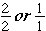. The ratio of 6 inches to 3 feet could be written as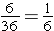. The ratio of four eggs to two dozen eggs could be written as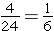eggs.

In your vocabulary words, we see that a proportion is an equation that states that two ratios are equivalent. There is more than one strategy for finding equivalent fractions and solving proportions. First, you can use the Identity Property of Multiplication.can be solved asTo check your work, simply cross multiply to confirm that these ratios are proportional.

7 * 96 = 672 and 12 * 56 = 672

Let’s look at the same problem and cross multiply to set up an equation to find x.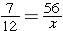Cross multiply to get 7 times x and 12 times 56. Write this as an equation.

7x = 672 Now, simply solve this equation by dividing both sides by 7.   x = 96.

There will be times when using the Identity Property of Multiplication will be the simpler solution, but when numbers are easily factored, cross multiplying to find the missing value, or cross multiplying to check for proportionality is the best way to go. Just remember to use your units of measure appropriately as you solve these types of ratio problems.

Using the same format, ratios of corresponding sides of similar figures can be used to check for proportionality and to solve for missing side lengths. Remember that similar figures have the same shape, where corresponding angles are congruent and corresponding sides are proportional. Take a look at the figures below.It appears that these two figures are similar shapes, meaning their angles are equal and their corresponding sides are proportional. Corresponding sides are sides in different polygons that are in the same relative position. In the figure above,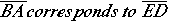. These sides are in the same relative positions in two different polygons. Also,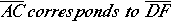. We can use this information to write ratios to check for proportionality. If these corresponding sides are proportional, then it looks as though we have similar shapes. Simply substitute side lengths for the side labels make sure that you set up the proportion correctly.

BA = 4 and ED = 8, so write the ratio as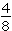.

AC = 10 and DF=20, so write the ratio as.

Now, write these ratios as a proportion and cross multiply to check for proportionality.; 4 * 20 = 80 and 8 * 10 = 80, so the shapes are proportional.

NOTE: You can also simplify each ratio and get– this also shows that these are proportional side lengths creating similar shapes.

Using the same process, you can find missing side lengths from similar shapes.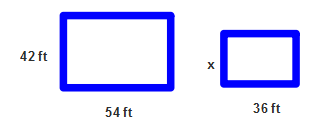First identify the corresponding sides and set up a proportion.Now, cross multiply and set up an equation

54x = 1512

Divide both sides by 54 to find x.

x = 28. The missing side length is 28.

You can also simplify 54 over 36 prior to cross multiplying.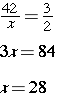Now that you have seen how to write and solve proportions with ratios and corresponding side lengths, we need to look at proportion word problems and how to set those up. Check out the following example.

A five-pound pack of flour costs \$1.80 and contains 12 cups of flour. Rachel is making cookies where the recipe calls for 2 cups of flour. Determine the cost for the flour needed for the cookies. Set up a proportion to solve the problem.

Set up the problem as you read. \$1.80 is your first number and corresponds to 12 cups of flour.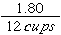and the next number you see corresponds to the denominator in your first ratio, so 2 will be the denominator in your second ratio;.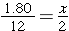. Now, cross multiply and set up an equation.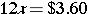x = \$.30 (2 cups of flour will be valued at 30 cents).

## Solve Proportions Practice

Set up a proportion for each word problem and solve.

1. Mrs. Johnson drove 105 miles in two and a half hours.   What was Mrs. Johnson’s speed in miles per hour?
2. A restaurant charges a single price for its buffet. The total bill for a table of 6 having the buffet was \$294. Each of the 8 people at a second table had the same buffet. What was the total bill at the second table?
3. On the Milestone test, Jacob answered the first 20 questions in 5 minutes. There are 76 questions on the test. If he continues to answer questions at the same rate, how long will it take him to complete the test from start to finish?
4. Will knows that a 45-ounce pitcher can hold enough lemonade for 6 people. At this rate, how many ounces of lemonade will he need to serve 26 people?
5. Two rectangular desks are similar. The larger one is 42 inches long and 18 inches wide. The smaller one is 35 inches long. What is the width of the smaller desk?
6. The official size of a basketball court in the NBA is 94 feet by 50 feet. The basketball court in the school gym is 47 feet long. How wide must it be to be similar to an NBA court?

(source)